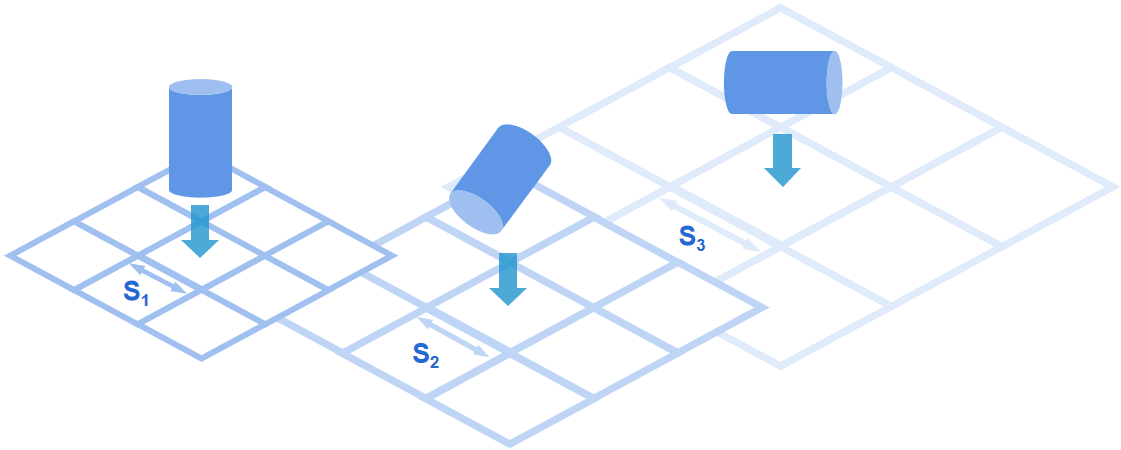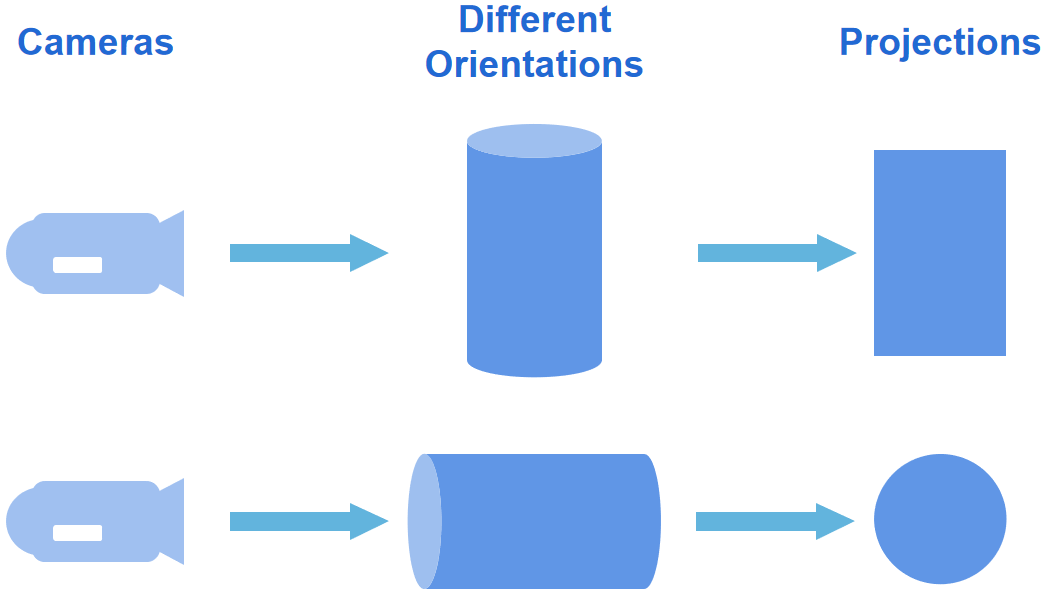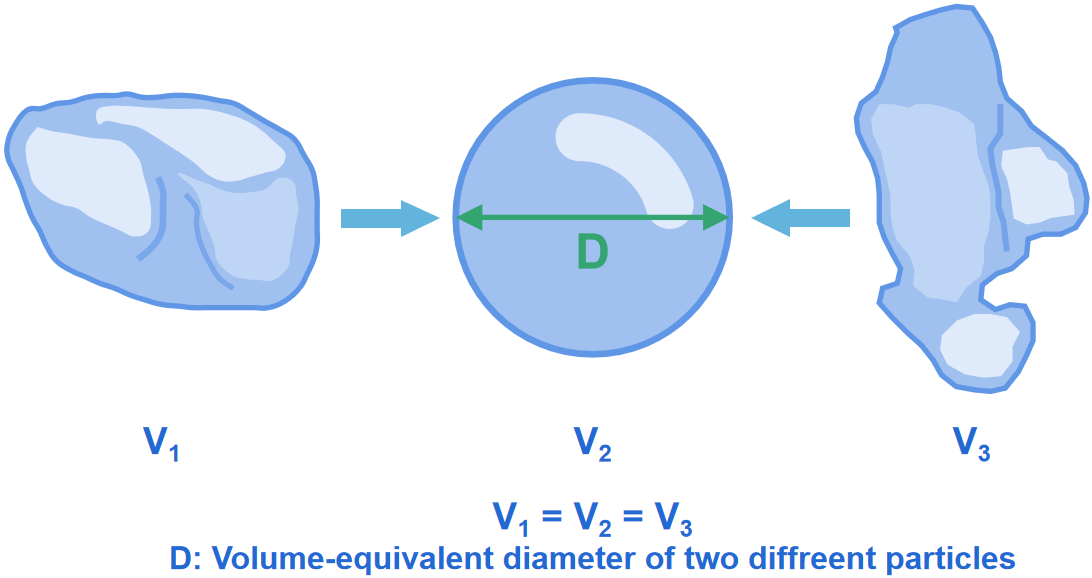Hmoe > Learn > Wiki > What is equivalent spherical diameter?

# What is equivalent spherical diameter?

2023-07-12WIKI

In most cases, the shape of the particle measured is irregular, which is not beneficial to communication on data, statistics, and analysis when using different measurement methods. Only perfectly spherical, non-porous, smooth, non-charged, mono-dispersed particles will give the same result on all techniques. Hence, the principle of an equivalent spherical diameter was introduced. The equivalent spherical diameter of an irregularly shaped particle is the diameter of a sphere whose physical, optical, or electrical property is the same as that of the particle. As examples, some typical equivalent spherical diameters are shown below.

## Sieve-equivalent diameter:

The sieve-equivalent diameter of a particle is the diameter of a sphere, which passes through a specific sieve aperture. For a sphere, the S1 diameter would be the same diameter as the sieve-equivalent diameter. However, if the particles were not perfectly spherical, the orientation of the particle affects the sieve-equivalent diameter significantly. As shown below, the sieveequivalent diameter varies due to different falling orientations.## Area-equivalent diameter:

The area-equivalent diameter of a particle refers to the diameter of a sphere whose projected area is the same as that of the particle. Area-equivalent diameter is commonly used in image analysis, in which the particle images are captured in random orientations, leading to different area-equivalent diameters of the same particle. However, results are obtained by averaging a large number of measurements, avoiding the impact of accidental errors.## Volume-equivalent diameter:

Particle size analysis based on laser diffraction technology commonly applies volume-equivalent diameter to present the particle size distribution. The laser diffraction equivalent diameter, which is a more accurate description, is calculated based on the scattering pattern of laser light as it is irradiated by the sample particles. However, the terms “volume equivalent diameter” and “laser diffraction equivalent diameter” are often used interchangeably, representing the equivalent diameter calculated based on the scattering pattern.## Stokes diameter:

Stokes diameter of a particle is the diameter of a sphere whose density and settling velocity are the same as those of the particle. Stokes diameter is also named equivalent spherical settling diameter. When particle size analysis based on gravitational and centrifugal sedimentation is carried out, measurement results using Stokes diameter are preferred.Struggling to grasp particle analysis concepts? Our new Beginner's Guide to Particle Analysis is here to help you grasp these concepts in the most accessible manner possible. Download it as a PDF to read on the train, on the plane, or wherever else there’s no internet.